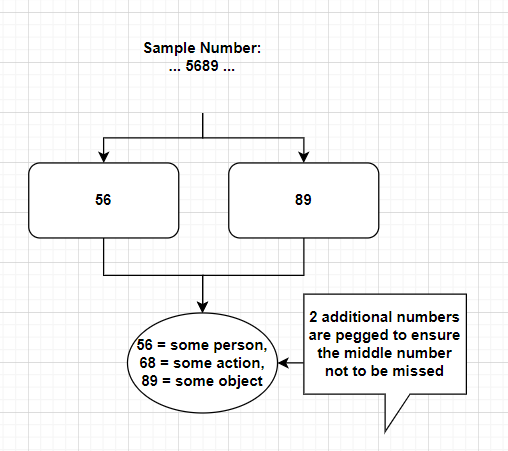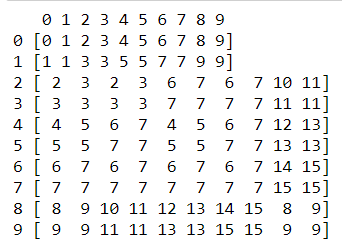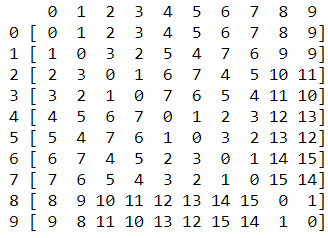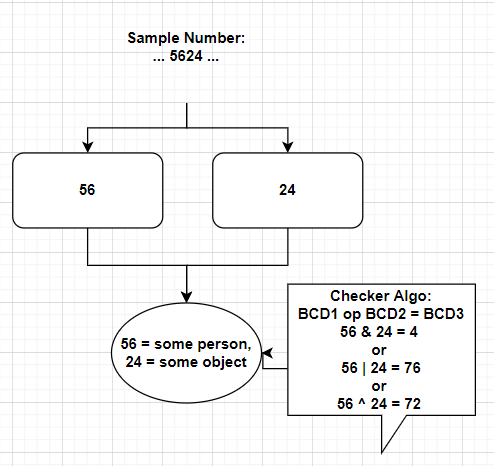# Checksum algorithm for large scale number memorization

It’s well-known that missing a certain part of memory is a discouraging issue in the memorizing process. The most straightforward way is to do spaced repetition.

Still, it’s a bit tedious if there are often missing images.
If we can chain our pictures stronger, then we won’t lose the image easily. One approach is by remodifying memory encryption.

An inspiration from pi-memory-athlete, using the double link to minimize the errors. An example with the PAO system.The advantage is each number are pegged twice, missing a digit you still have roughly 50% to get it correct (encrypted twice for a digit). The drawback is inefficient, compression rate of 3:1 (3 pegs for a two-digit number).

How to obtain better efficiency?
Potential of bitwise operation!

1. and operation
2. or operation3. xor operationHow checker algo applies.The checksum generated from operation “and” and “or” either way cause too much data loss, thus not suitable. e.g. 00 and 11 = 00; 00 or 11 = 11

The compression rate of the algo 50% and the accuracy is not too bad, might be over 66%.
For checksum algo 3, using the inverse property xor, we know that A xor B = C, C xor A = B or C xor B = A. Perfect!

However, to realize such checksum algo, you need to familiar with xor operation. Luckily, it’s not quite difficult. Here, is the way of how I memorize it, though, you can always do it in the traditional way.

PS in 1 xor? the line should be [odd-, even+]
example of cross pair property.The hardest challenge is that bc of PAO is 100 images per set, you need to update with O from 100 pegs to 256 pegs so as to make this method works.

Every method has its advantage and drawback, I hope my little contribution brings a more innovative idea to the community.4 Likes

I cast out nines to check. Not fool proof. If you are off by a multiple of 9 it won’t detect it, but it’s fast and 90% effective.

3 Likes

how to do so, can you explain it more briefly, thank you!

“Casting out nines” is an ancient method of checking calculations by checking the remainders after division by 9. This can be done very fast by the trick of adding the digits in any order, replacing 9 by zero every time the sum exceeds 9

Eg 12345 mod 9 = 6 since 4+5 = 9 => 0

The modulus is preserved in arithmetic operations.

28 * 79 = 2212 the remainders are 1 * 7 = 7

This gives a quick way to check calculations to within a multiple of 9.

There is also scheme based on 11 which is a little more complicated

1 Like

Great idea for mental calculation, butwhat’s the relationship between cast 9 and memory checker?

For instance, if u r told to remember a dataset, one group numbers are 2879. Then u did cast out nines, can u demo when do u know u remember it correctly or wrong? Also, given that you remember ur checksum, can u find out ur missing digit? I look forward to hearing the solution,thanks.

Simply memorize the mod 9 value for every, say, 10 digits and then cast out 9s on what you recall to check.

1 Like